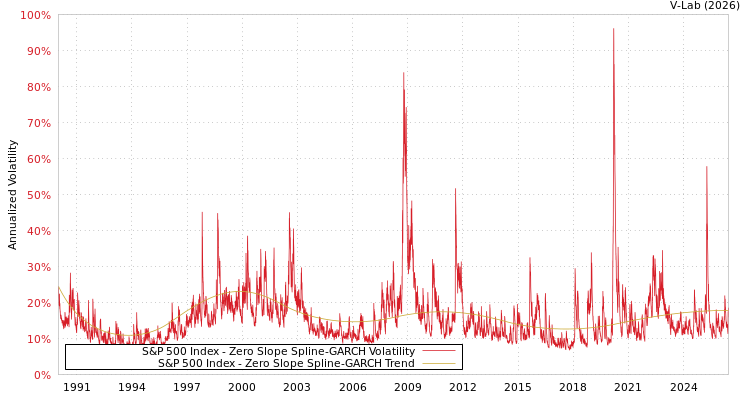##### S&P 500 Index Zero Slope Spline-GARCH Volatility Analysis
###### Volatility Prediction for Monday, October 25th, 2021:12.24% (-0.57%)

Analysis last updated: Friday, October 22, 2021, 09:48 PM UTC

###### Date Range:

from

to

6M ·

1Y ·

2Y ·

5Y ·

10Y ·

Allparamt-stat
$\omega$1.05886.76
$\alpha$0.10219.06
$\beta$0.854758.85
${\gamma }_{1}$-0.0001-0.00
${\gamma }_{2}$0.10021.25
${\gamma }_{3}$-0.1726-3.17
${\gamma }_{4}$0.02120.41
${\gamma }_{5}$0.17433.48
${\gamma }_{6}$-0.2382-5.39
${\gamma }_{7}$0.15473.09
${\gamma }_{8}$-0.0124-0.23
${\gamma }_{9}$-0.0451-1.04
Estimation Period:
Jan 1, 1990 to Oct 22, 2021